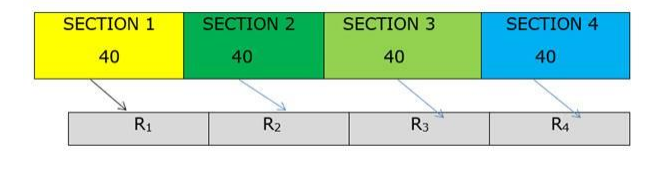# In a school, there are four sections of 40 students each in XI standard.

Question:

In a school, there are four sections of 40 students each in XI standard. In how many ways can a set of 4 student representatives be chosen, one from each section?

Solution:

Given: there are four sections of 40 students each in XI standard.

To find : number of ways in which a set of 4 student representatives be chosen, one from each section.

Ways of selecting one student from section $1:{ }^{40} \mathrm{C}_{1}$

Ways of selecting one student from section $2:{ }^{40} \mathrm{C}_{1}$

Ways of selecting one student from section $3:{ }^{40} \mathrm{C}_{1}$

Ways of selecting one student from section $4:{ }^{40} \mathrm{C}_{1}$So number of ways of choosing a set of 4 student representatives one from each

section $={ }^{40} \mathrm{C}_{1} \times{ }^{40} \mathrm{C}_{1} \times{ }^{40} \mathrm{C}_{1} \times{ }^{40} \mathrm{C}_{1}$

= 40 × 40 × 40 × 40

= 2560000